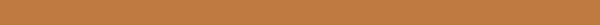# QNA: Computational Condensed matter

## Modified: 2020/05/21

### Can I ignore the small imaginary frequencies in phonon spectrum calculation?

The full question coming from the daily works when calculating the phonon diagram. To make sure that the structure is dynamic stable at the relaxed configuration, the phonon diagram is a useful tool to proof this kind of stability. However, when the result coming with the undesired imaginary frequencies, can we simply ignore it to say that our configuration is at its stable phase? Or we have to dig into the details of the causing imaginary frequencies? And how small is small enough to ignore these imaginary frequencies? Most real life occurring question when using frozen phonon method (such as phonopy [^phonopy] by Prof.Togo) is that can we eliminate the imaginary frequencies by extending the supercell?

To answer this question, we should firstly have the physical intuitive of what is vibrational frequency in periodical systems and what is the source of imaginary phonon frequencies.

Imaginary frequencies can be represented in the same diagram as their positive counterpart, so they are also called ‘soft modes’. The reason such modes appear can reasoned either from a) numerical error in the calculation or b) a pathway for a symmetry lowering phase transition.

To exclude that imaginary frequencies caused by calculation errors, we can check the mode by extract it and perform static calculations along a trajectory of phonon displacement vector. The energy will reduced if the the mode is actually unstable. Whiles the energy will increase if the imaginary frequencies are caused by numerical errors.

If it is not the numerical error caused unstable modes, we need dig into very details of how that unstable factors happened. Here, we jump into the last question i.e. when using frozen phonon method can we eliminate the imaginary frequencies by extending the supercell? The direct answer to this question from my personal point of view is if the crystal possesses a long interaction range, then the fake soft mode for acoustic modes in vicinity of $\Gamma$ points is caused by missing force constance to the far away atoms. At this point, increase of supercell size can resolve this problem.
This problem will not happened if we use the DFTP method where we do not use supercell size as a variable.

Additional to the long interaction range, there are some non-negligible reasons which may be the source of soft modes[^softmode] no matter we use frozen phonon or DFTP methods:

• If the crystal is magnetic, and there exists some phonon-magnetic coupling, the phonon dispersion curves may depend whether one treats the crystal as non-magnetic, or ferro-, or antiferromagnetically.
• If in the crystal exhibits strong electron correlation, then the electronic band structures, and phonons must be calculated within the LDA+U, or GGA+U approach.

Some more important things need to be noticed when to find the stable transitional phase from the one with imaginary frequencies. We should find these structures by using supercells which have the same symmetry of the searched low symmetry phase.